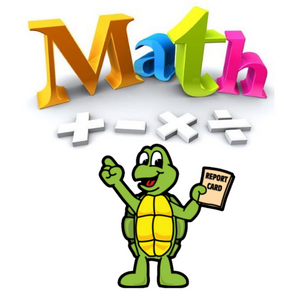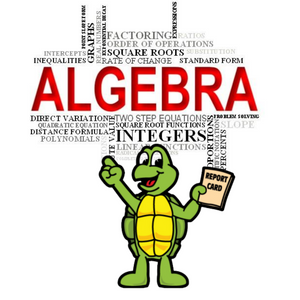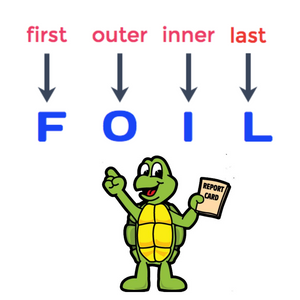# Free Resources

## Our MissionAt First Addition Tutors, our mission is to "fix" the disconnect of a student's dislike for mathematics. Instead of hating math, we individually work on building an appreciation and fascination of the beauty of mathematics. Once that is built, the walls can be broken down. When our students have an appreciation of math and see how simple math can be, that is when we all win!Simple: 1) We love math 2) We love to teach.

## The Basics- AlgebraThe purpose of learning math is to expand ones' logical mind, to see patterns, to be able to better problem solve. Algebra is the very basics of mathematics. Algebra may sound scary, but it is simple and beautiful. If we have 2 chocolate bars and we need a total of 5, how many more chocolate bars do we need? We can set up a variable, c, for the number of chocolate bars.  2 + c = 5. We can simply solve this equation and find out that we need 3 more chocolate bars for this equation to be true.  There...